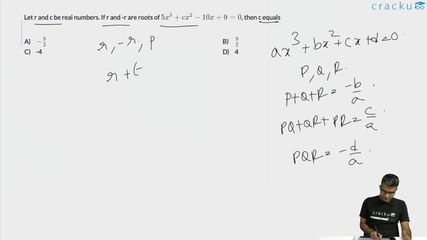Question 51

# Let r and c be real numbers. If r and -r are roots of $$5x^{3} + cx^{2} - 10x + 9 = 0$$, then c equals

Solution

Let the roots of the given equation $$5x^{3} + cx^{2} - 10x + 9 = 0$$ be r, -r and p

r - r + p = $$-\frac{c}{5}$$

p = $$-\frac{c}{5}$$ ...... (1)

$$-r^2-pr+pr=-2$$

$$r^2=2$$ ...... (2)

$$-r^2p=-\frac{9}{5}$$

$$p=\frac{9}{10}$$ ...... (3)

Substituting p in (1), we get

$$\frac{9}{10}=-\frac{c}{5}$$

$$-\frac{9}{2}=c$$

### View Video Solution